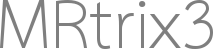# Mrresize 4D data to 3D

dear all,
i need to resize a 4D diffusion data to 3D diffusion data, i used the instruction of mrresize but i have an error, i will appreciate any help, thanks.

Have you tried mrconvert?

you can trying something like this…
mrconvert 4D_data.mif -coord 3 0 - | mrconvert - 3D_data.mif -axes 0,1,2

I’m sure there are other ways of converting 4D to 3D.

Cheers,
Sam

1 Like

You could do this in a single `mrconvert` call if you want:

``````mrconvert 4D_data.mif -coord 3 0 -axes 0,1,2 3D_data.mif
``````

But more to the point:

What do you mean by that exactly? If you have 4D data, converting that to 3D means extracting one volume from the 4D series (which is what the above call does), or it could refer to processing the data to generate a 3D output (e.g. DTI fit to obtain FA or MD, …). What do you mean exactly?

• If you mean to extract the b=0 volume, then the above will do the job most of the time, but it would be safer to use `dwiextract -bzero` (potentially followed by `mrmath -axis 3 mean` if there’s more than one).

• If you mean to extract tensor metrics, then you’d need to use a combination of `dwi2tensor` and `tensor2metric` like this:

``````dwi2tensor dwi.mif - | tensor2metric - -fa fa.mif -adc md.mif
``````

or variations thereof.

• If you mean something else, then you’ll need to be more specific as to what you’re actually trying to achieve.

1 Like

when will be the right time to run this command before running dwipreproc or after that?

I tried converting the data to 3D using the by using the above mentioned command but it is asking only for the 4D data. I don’t when to run

mrconvert 4D_data.mif -coord 3 0 -axes 0,1,2 3D_data.mif

Ok, maybe the order of operations isn’t what I thought it was… If it doesn’t work, then your suggestion should indeed do the trick…Dear Tournier,
Tracks are still the same after running the below command

mrconvert 4D_data.mif -coord 3 0 -axes 0,1,2 3D_data.mif

I tried changing the pipeline for the track generation it’s still the same. I am still searching for the reason for these types of tracks.

i need to resize a 4D diffusion data to 3D diffusion data,

What do you mean exactly?

Third option: One way to “map” four-dimensional data to 3D is to compute some statistic across volumes; so for instance, if you have a few b=0 images in a 4D series, and compute the mean value across volumes via `mrmath -axis 3`, you end up with a 3D image.

But as it stands currently, it is entirely unclear to us based on your current description exactly what you are actually trying to achieve; hence more questions than answers. We typically wouldn’t describe producing a 3D dataset from a 4D dataset as “resizing”, as there by necessity must be some kind of explicit operation that results in that change in dimensionality, rather than the change in dimensionality being the goal in and of itself.

2 Likes

Dear all,
thanks for your responses, i have solved the problem with the command
mrconvert 4D_data.mif -coord 3 0 -axes 0,1,2 3D_data.mif